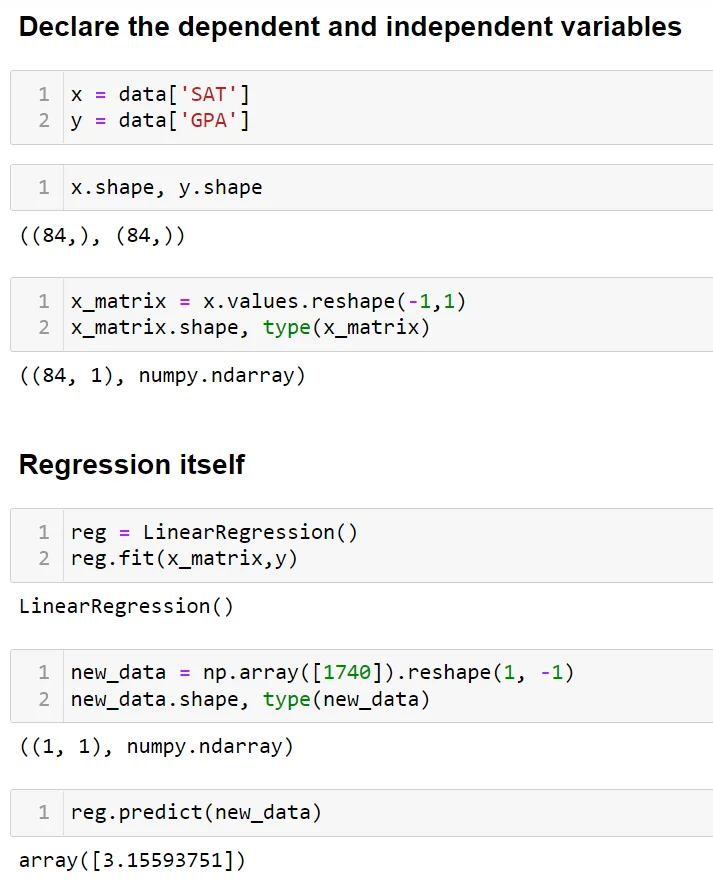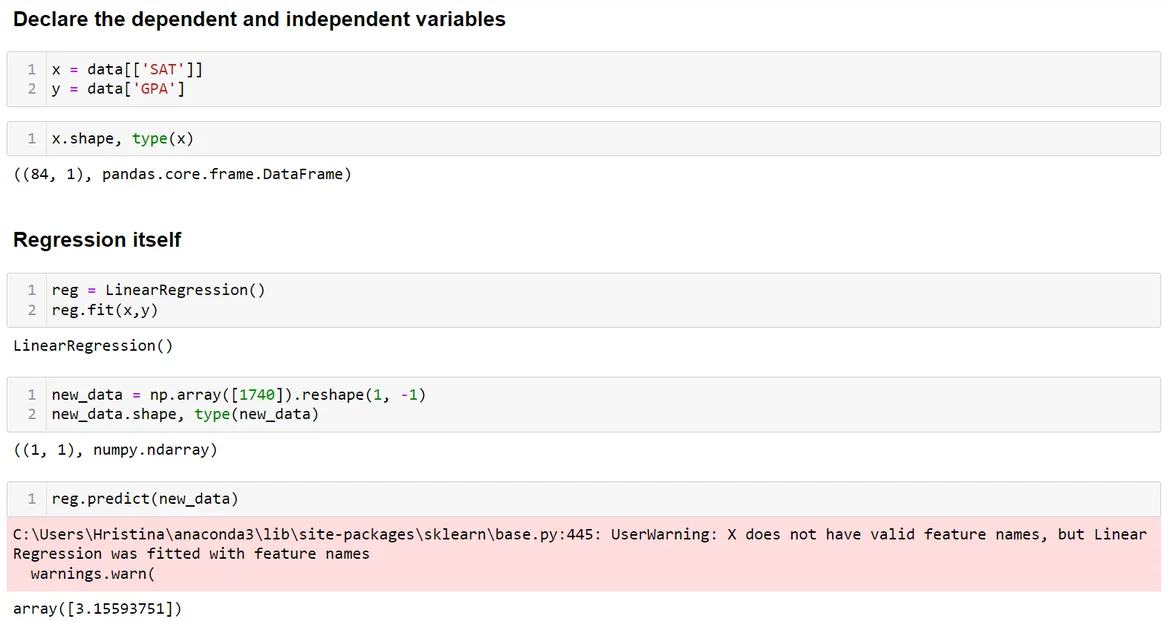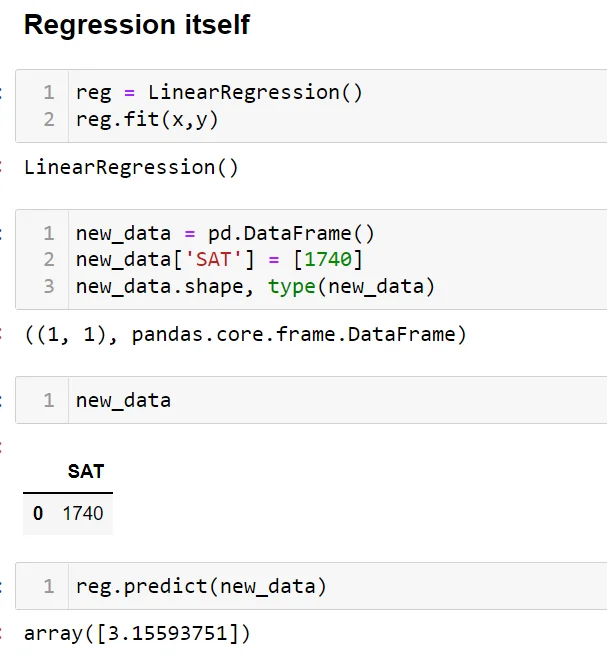30 Jun 2022

Posted on:

29 Jun 2022

0

# Can I use "x = data[['SAT']]" instead of "x = data['SAT']",?

When I try "x = data[['SAT']]" (as we did in multiple regression) instead of "x = data['SAT']", it seems to work fine without having to reshape the data; and I get the same results (e.g., intercept and coeffs). Is there any particular advantage of reshaping the data here?

Thank you.

Instructor
Posted on:

30 Jun 2022

2

Hey,

Thank you for the great question!

``````x = data[['SAT']]
x.shape
``````

and the solution in the lecture

``````x = data['SAT']
x_matrix = x.values.reshape(-1,1)
x_matrix.shape
``````

will return a variable of shape `(84, 1)`. This means that both approaches will work without an error when performing the regression, but we need to take some caution here. Let me demonstrate this with an example.

Let's first look at the version of the code where we use `x = data['SAT']` and apply the `reshape()` method.The variable `x_matrix` is of shape `(84, 1)` and of type `numpy.ndarray` (that is, an n-dimensional array). The simple linear regression, therefore, runs without any errors. The subtlety comes next, when we want to predict the GPA, given an SAT score. We need to make sure that the variable `new_data` has the same number of columns and is of the same type as the variable we put in as a first argument in the `fit()` method - in our case, `x_matrix`. Indeed, `new_data` has 1 column, representing the SAT, and is of type `numpy.ndarray`. The `predict()` method runs flawlessly.We see that the shape of the `x` variable is indeed `(84, 1)` but the type is different. It is a `DataFrame`, rather than an `ndarray` (try and print it out to see what the `x` variable stores). The `fit()` method works fine, as expected. Let's now try and predict the GPA for an SAT score of 1740, defining `new_data` in the known way. We do get a result, but also a warning. What it tells us is that `new_data` doesn't have valid feature names (as it is an `ndarray`) but the variable `x`, which we used to fit the model, does (as it is a `DataFrame` object). What we need to do is define `new_data` such that it also is a `DataFrame`, containing a single column called `SAT`.The warning is now gone.

To sum this up, you always need to make sure that the variable you use to make predictions is of the same type and has the same number of columns (arranged in the same way) as the feature matrix you use to fit the model.

To answer your question, there is no particular advantage of reshaping a matrix over directly using the `DataFrame` object, so long as you are consistent throughout your code.